Categories

# ECON1064: Quantitative Analysis Question 1 The below graph plots the daily prices of Gold over time. a) Examine the plot and describe the issues that may arise when trying to forecast future Gold prices given this time series data. Offer solutions to these issues. b) Offer a suitable forecasting method for this data.

 ECON1064: Quantitative Analysis

Question 1

The below graph plots the daily prices of Gold over time.

a) Examine the plot and describe the issues that may arise when trying to forecast future Gold prices given this time series data. Offer solutions to these issues.
b) Offer a suitable forecasting method for this data.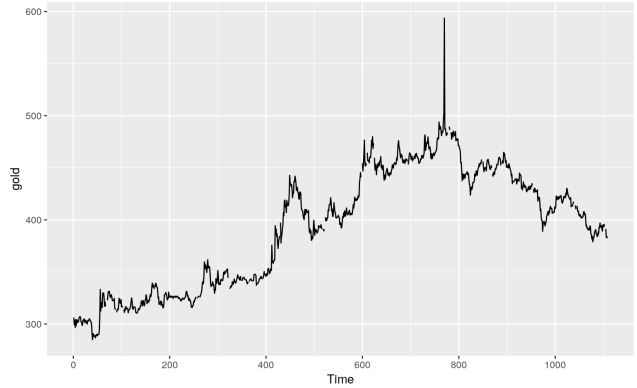Question 2

For each of the below ACF plots which are obtained for a time-series data of 4 different variables of interest:
a) Explain the ACF plot.
b) Describe what the raw data is likely to look like over time.
c) What kind of variable is this plot likely to characterize (e.g., stock prices, exchange rates, temperature, etc.)?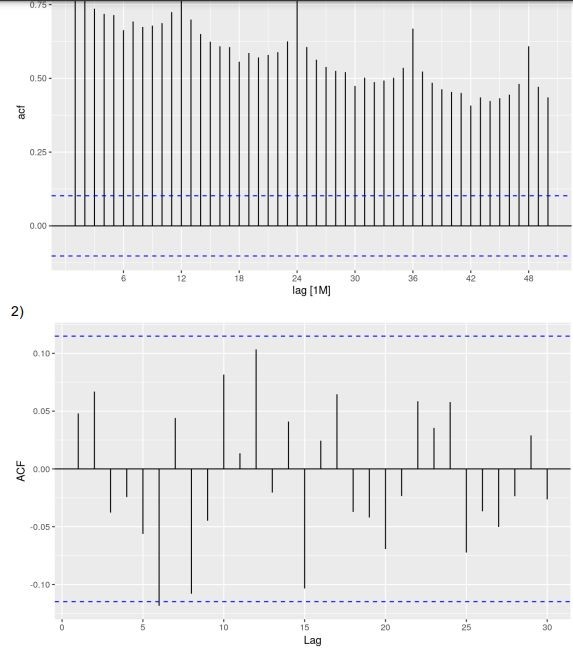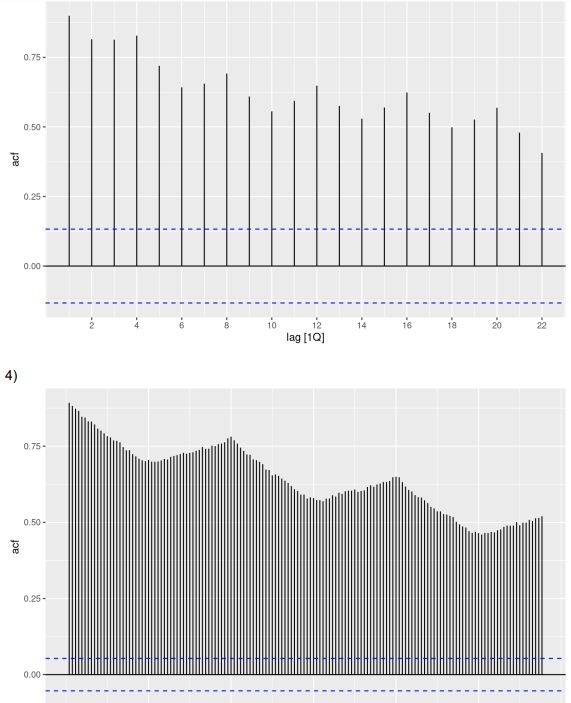Question 3

The following ACF plots were produced for raw data of monthly sales of two different variables, A and B.
a) Explain which variable is likely to be easier to forecast.
b) Explain how your answer to part a) would change if these were residuals of an ARIMA model instead of “raw data of monthly sales”.

Variable a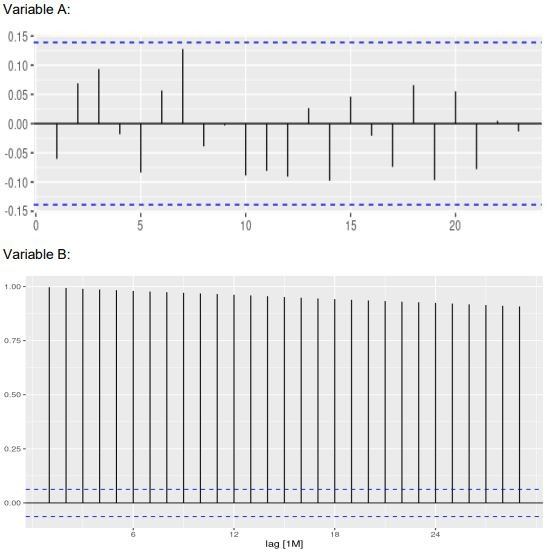Question 4

For the following time series plots, explain what type of transformation, if any, would make the variance more stable.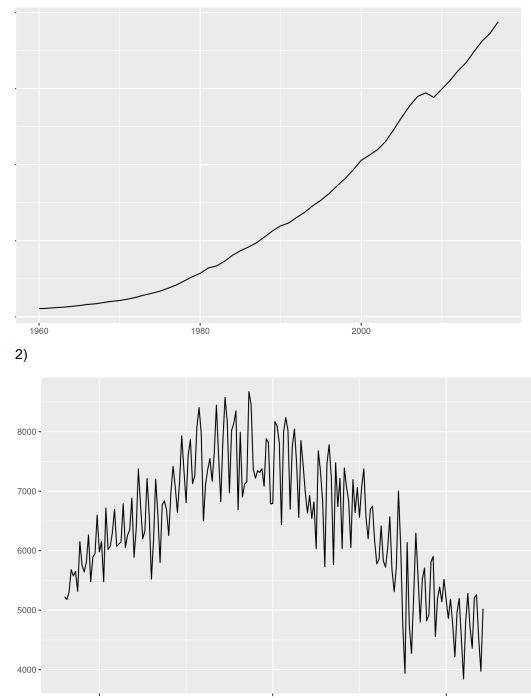Question 5

The following monthly sales (in thousands of AUS dollars) of chocolate boxes have been recorded for January, February, March, and April, respectively: 8, 8, 5, 9. Focusing on sales forecast accuracy for the month of April only, explain which of the following forecasting method would you recommend: the Naïve method, the Average method, or the Simple exponential smoothing method (assuming alpha=0.8 and the initial state of 7)?

Question 6

The variable income (yearly) is examined in a regression setting where the predictor variable is lag (1) of income and the following output is produced.
a) Write down the regression equation.
b) Interpret the meaning of the slope.
c) A dummy variable for gender (male=0, female=1) was added to the model. Interpret its coefficient of -0.2.

Question 7

The following plots have been obtained for a time series.
a) Suggest an appropriate ARIMA model.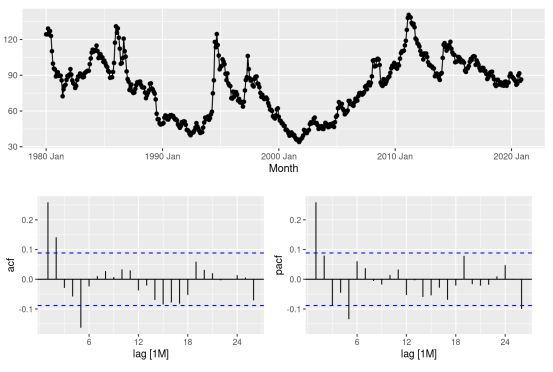b) The following ARIMA output has been obtained from R. Based on this output, which model would you recommend for forecasting?

c) If your selected model in part b) above has a p-value of 0.13 in the Ljung-Box test, would you recommend using this model? Explain why or why not.

Question 8

Examining the below R output, explain what model was selected for forecasting?